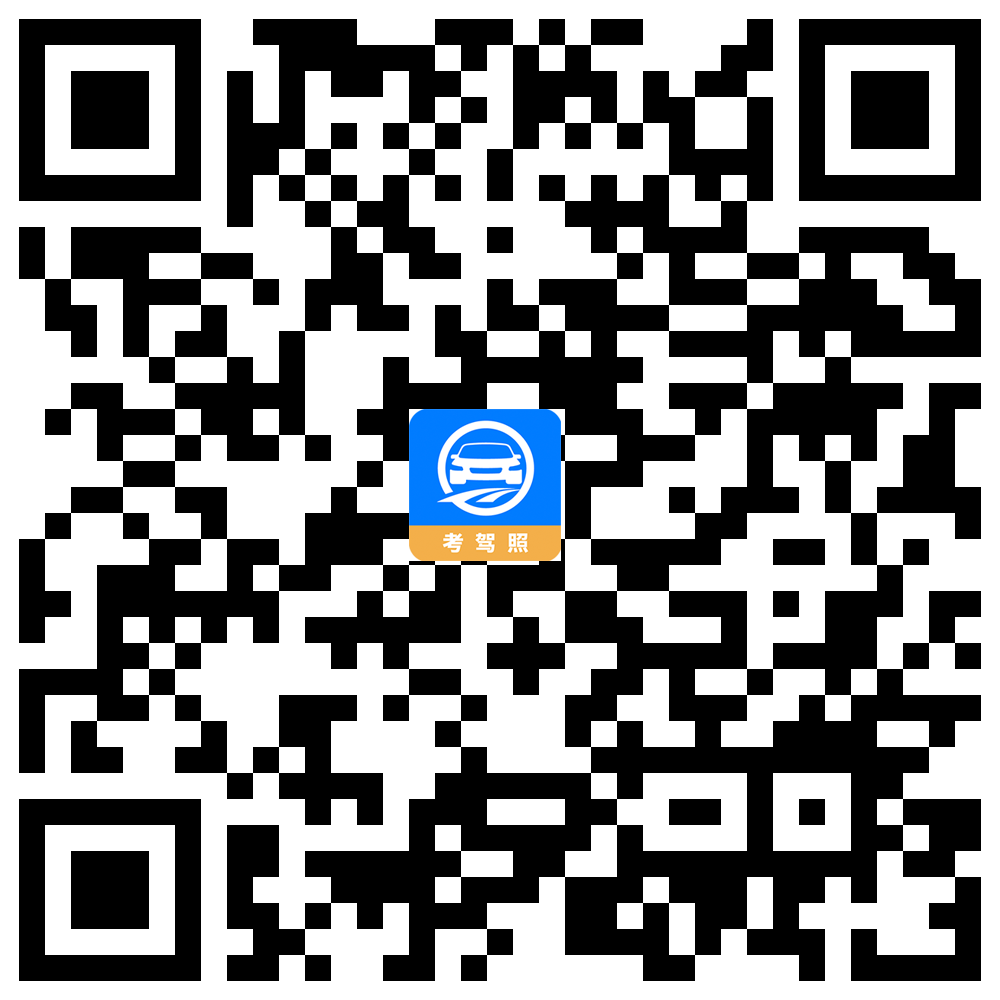564634598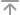返回顶部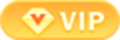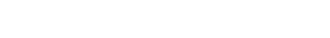## 警告标志

•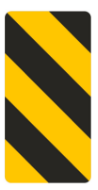右侧通行(新）

•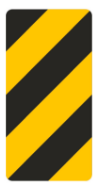左侧通行(新）

•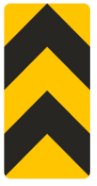两侧通行(新）

•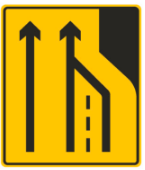车道数变少（新）

•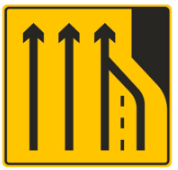车道数变少（新）

•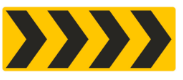线形诱导标(新）

•线形诱导标(新）

•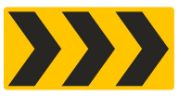线形诱导标(新）

•线形诱导标(新）

•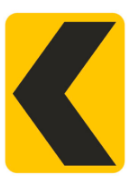线形诱导标(新）

•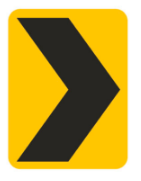线形诱导标(新）

•减速丘(新)

•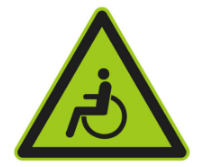注意残疾人（新）

•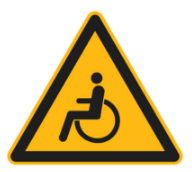注意残疾人（新）

•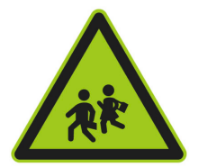注意儿童(新）

•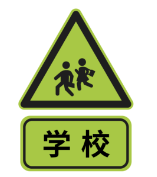注意儿童标志加辅助标志实例

•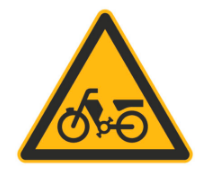注意电动自行车

•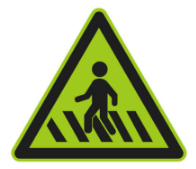注意行人(新）

•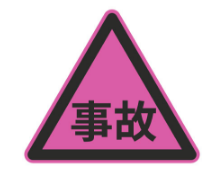交通事故管理

•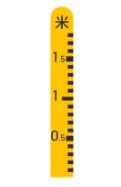注意积水

•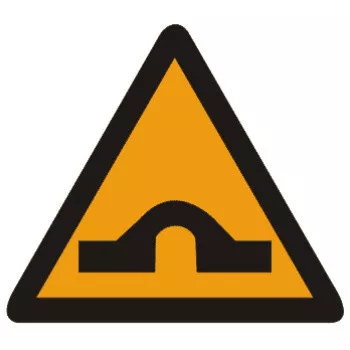驼峰桥

•隧道

•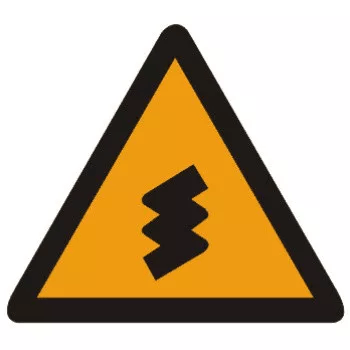连续弯路

•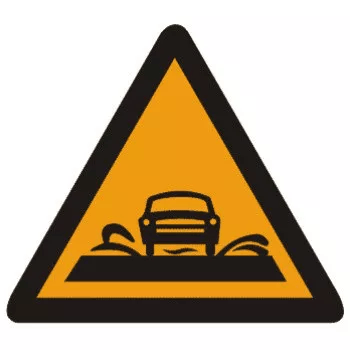过水路面

•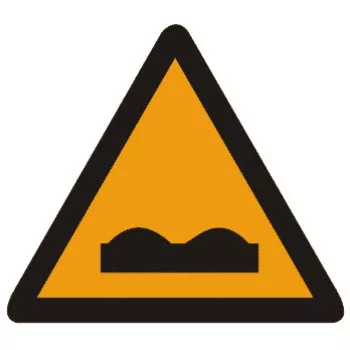路面不平

•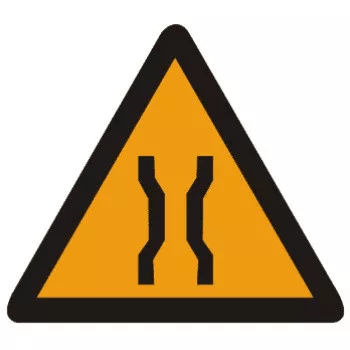窄桥

•环形交叉

•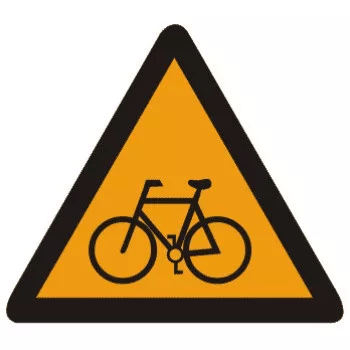注意非机动车

•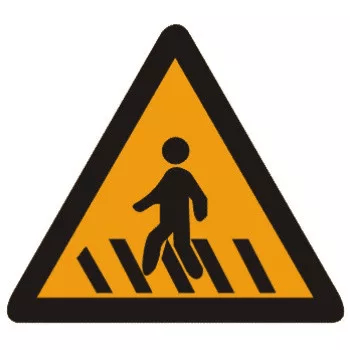注意行人

•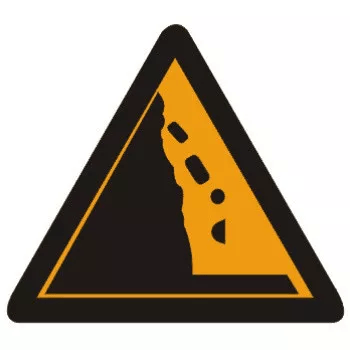注意落石

•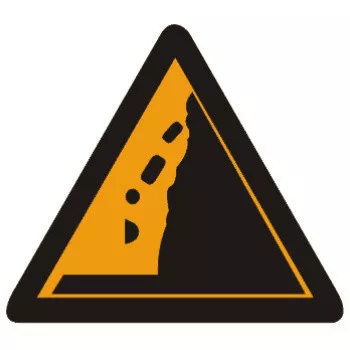注意落石

•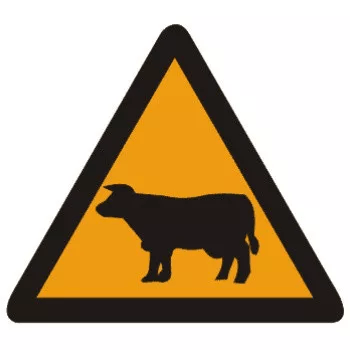注意牲畜

•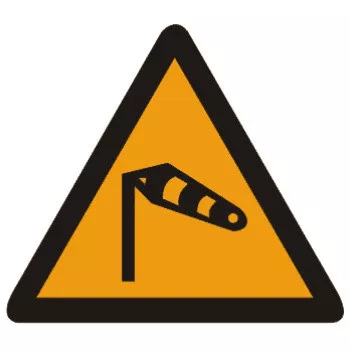注意横风

•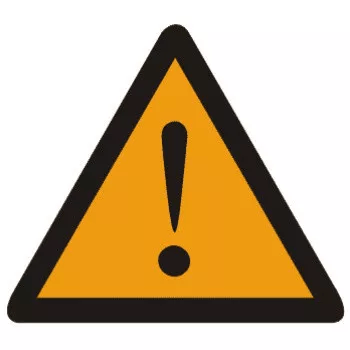注意危险

•注意儿童

•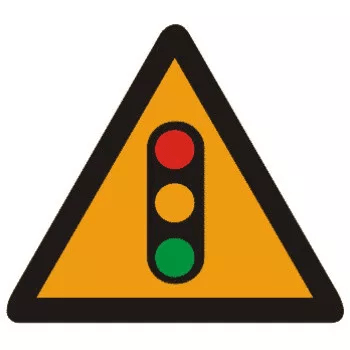注意信号灯

•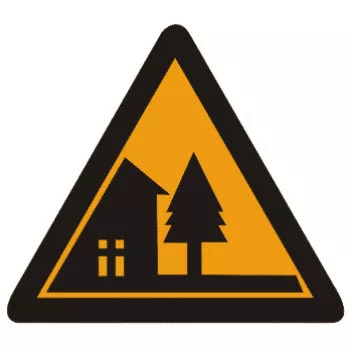村庄

•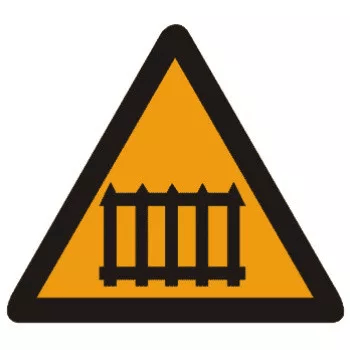有人看守铁路道口

•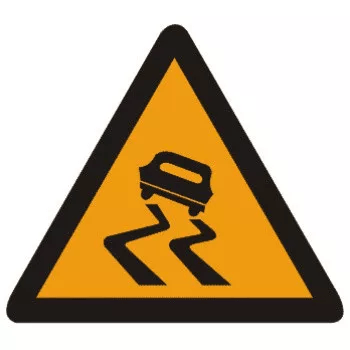易滑

•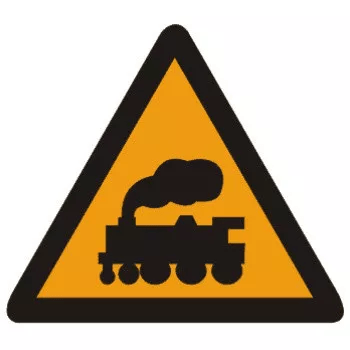无人看守铁路道口

•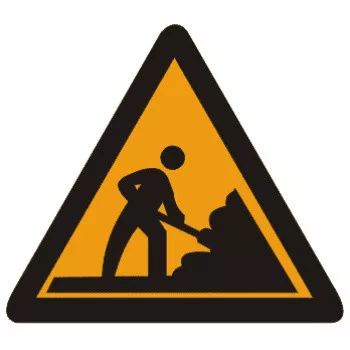施工

•斜杠符号

•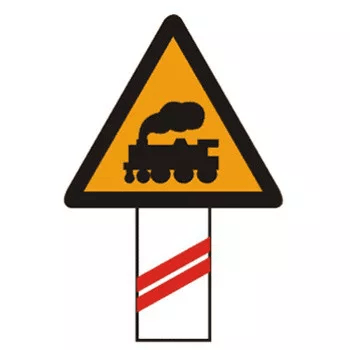斜杠符号

•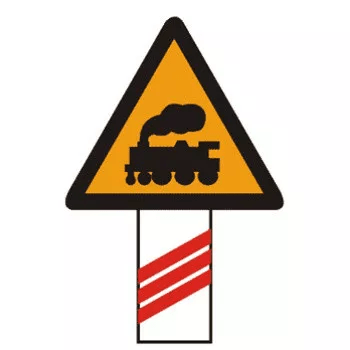斜杠符号

•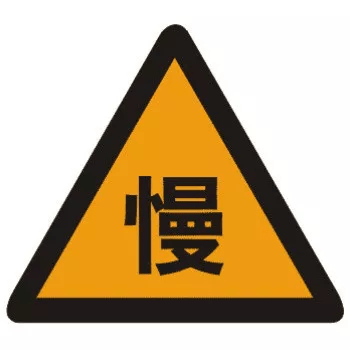慢行

•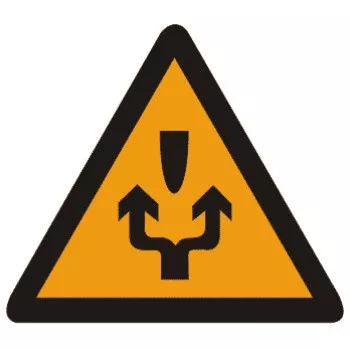左右绕行

•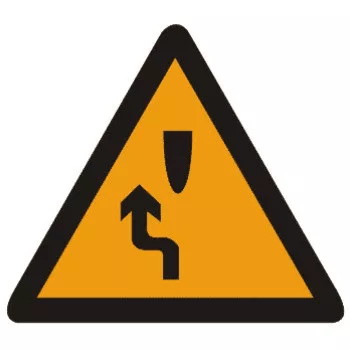左侧绕行

•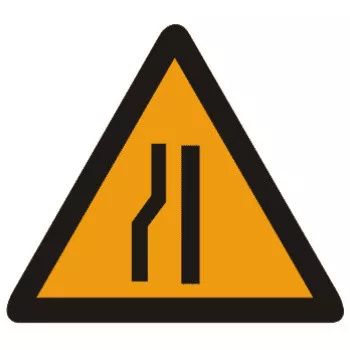左侧变窄

•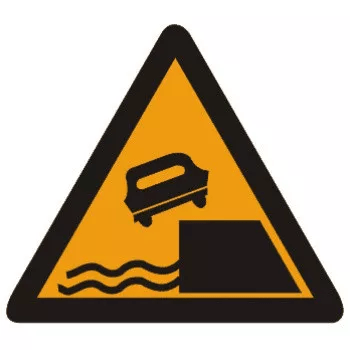堤坝路

•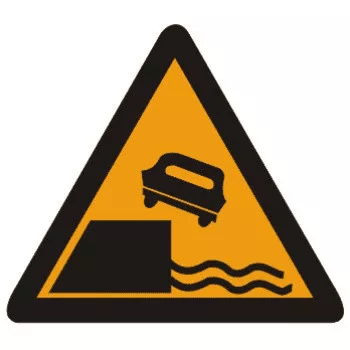堤坝路

•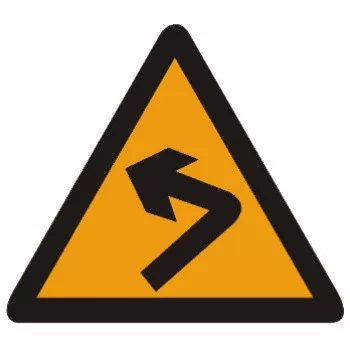向左急弯路

•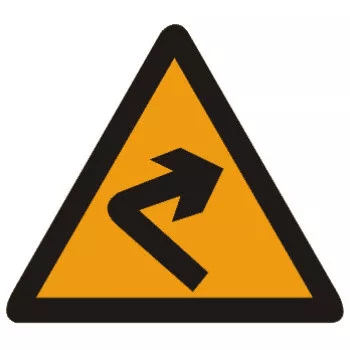向右急弯路

•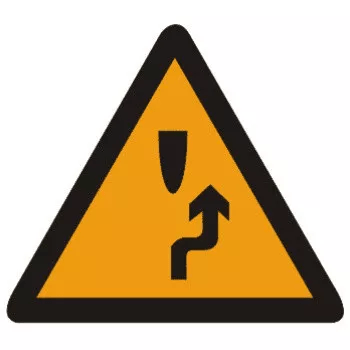右侧绕行

•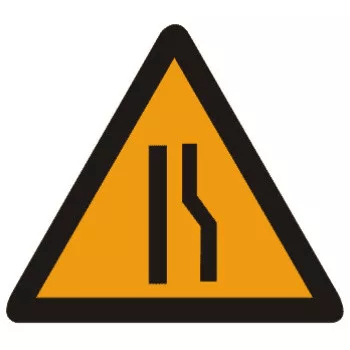右侧变窄

•反向弯路

•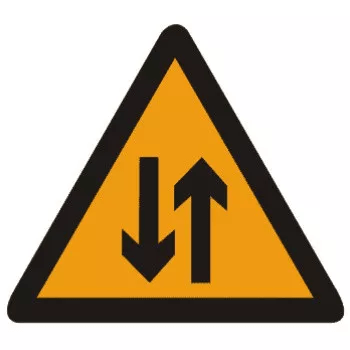双向交通

•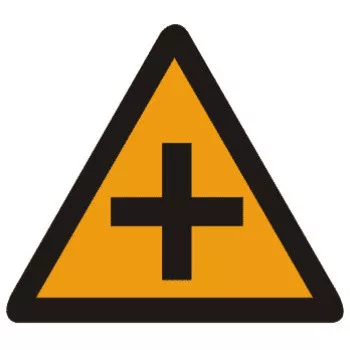十字交叉

•傍山险路

•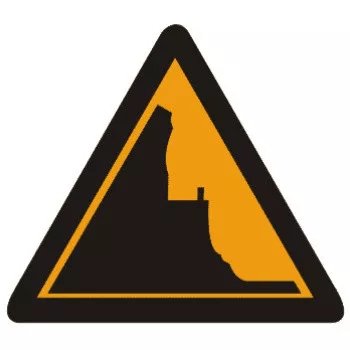傍山险路

•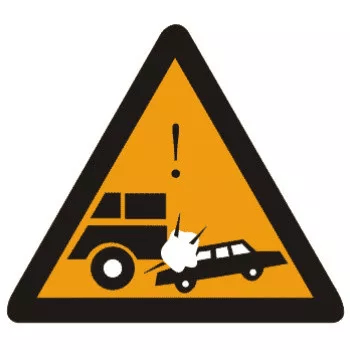事故易发路段

•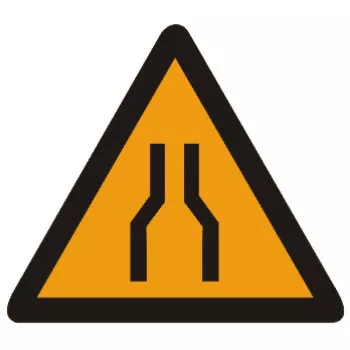两侧变窄

•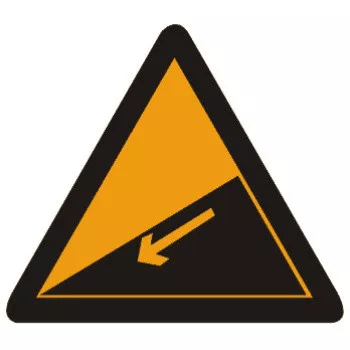下陡坡

•上陡坡

•Y形交叉

•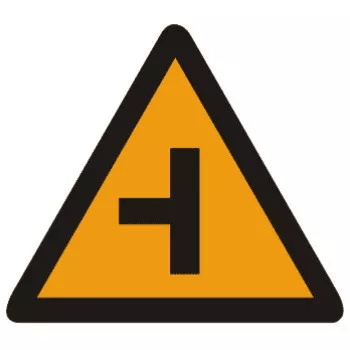T形交叉

•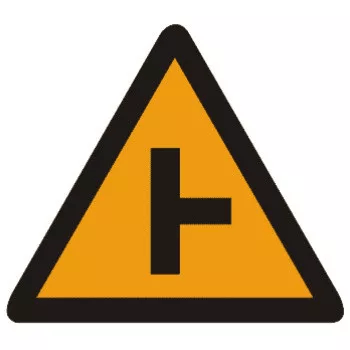T形交叉

•T形交叉